# C259 – AGA 7 & AGA 9 – Gas Flow Rate Calculation

## Description

This calculates the volume, standard volume or mass flow rate of a gas as specified in AGA 7 Appendix B. Note that AGA 9 refers to AGA 7 for these flow rate calculations.

AGA Report No. 7 – Measurement of Natural Gas by Turbine Meters

AGA Report No. 9 – Measurement of Gas by Multipath Ultrasonic Meters

Kelton calculation reference C259

## Options

### Input flow rate

• Volume flow rate at flowing conditions
• Volume flow rate at base conditions
• Mas flow rate

This option is used to select the input flow rate

### Calculate

• Volume flow rate at flowing conditions
• Volume flow rate at base conditions
• Mass flow rate

Two of these three options will be available for selection depending on the input flow rate

## Calculation

### Volume flow rate at base conditions

Depending on the flow rate input, volume flow rate at base conditions is calculated by: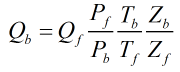or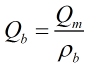### Volume flow rate at flowing conditions

Depending on the flow rate input, volume flow rate at flowing conditions is calculated by: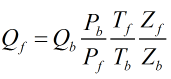or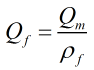### Mass flow rate

Depending on the flow rate input, mass flow rate is calculated by: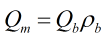or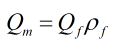Where Pf = Absolute pressure at flowing conditions Pb = Base pressure Tf = Absolute temperature at flowing conditions Tb = Base temperature Zf = Compressibility at flowing conditions Zb = Compressibility at base conditions ρf = Density at flowing conditions ρb = Density at base conditions

Back